# 如何把Java的double类型变量保留两位小数

``````import java.text.DecimalFormat;

public class Test {
public static void main(String[] args) {
String str = "1.00";
DecimalFormat df = new DecimalFormat("0.00");
double d = Double.valueOf(str);
double dd = 1.00;
double ddd = 1.01;
System.out.println(str);   // 1.00
System.out.println(str instanceof String); // true
System.out.println(d);  // 1.0
System.out.println(dd); // 1.0
System.out.println(ddd); // 1.01
System.out.println(df.format(d)); // 1.00
System.out.println(df.format(d) instanceof String); //true
}
}
``````

Java对double小数点后如果有两个0,只会保留一个吗？

0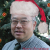``````import java.math.*;

public class A {
public static void main(String args[]) {
double f = 111231.558564;
System.out.printf("直接将双精度的变量 f, 按C语言的输出格式输出：%.2f\n",f);
BigDecimal b = new BigDecimal(f);
double f1 = b.setScale(2, BigDecimal.ROUND_HALF_UP).doubleValue();
System.out.println("f1 是小数点后只有两位的双精度类型数据：" + f1); // f1 是双精度类型
}
}  ``````

f1 是小数点后只有两位的双精度类型数据：111231.56

http://www.jb51.net/article/46010.htm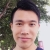0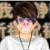String.format(),找找这个方法的用法，实在不行就自己实现吧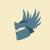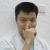0``````System.out.println(dd);